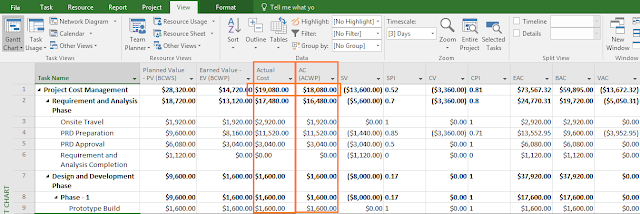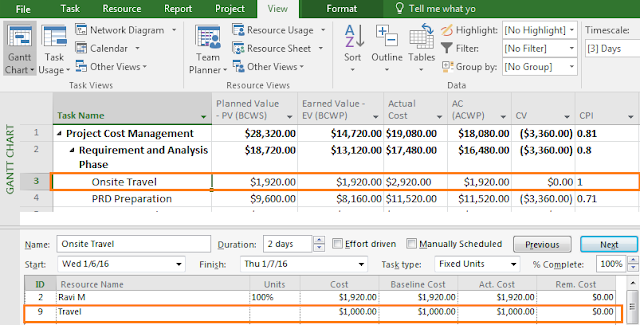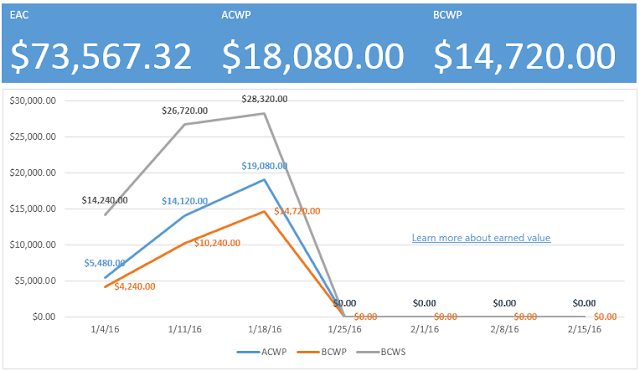## Sunday, April 03, 2016

### Actual Cost Vs Actual Cost of Work Performed(ACWP) - Practical PMP with MS Project

In a recent session of Practical PMP with MS Project, conducted globally, one of the participants had a problem while calculating the EVM metrics.

I. The Issue

The project was planned and then baselined.  Post it, the execution has been undergoing (we were in Cost Management knowledge area). To check on the project variances, status date was set. After tracking was done with MS Project, actual data got populated. Next, when he tried to calculate Estimate to Complete (ETC), a metric in Earned Value Management (EVM), there was a mismatch while using the Actual Cost (a field in MS Project) as compared to using AC(ACWP) field.
He was not sure which field ETC should take for its calculation - ACWP or Actual Cost. MS Project 2016 was used in the session.
Do note that ETC metric of EVM is not available by default in MS Project. You have to create a custom field and apply formulas to get ETC. Other metrics such as VAC, BAC etc. are available by default.

Here is a snapshot of the project that the participant had done.Figure - 1 - EVM Metrics
As you can see, the Actual Cost and AC(ACWP) field have different values. Actual Cost is \$19,080 whereas AC(ACWP) is \$18,080! This raised questions.

Is there anything that went wrong in calculation?
Which field is considered for Cost Variance? Is it Actual Cost or AC(ACWP) field?
Which field is considered for Cost Performance Index (CPI)? Actual Cost or AC(ACWP) field?

II. A Brief Theory on EVM Metrics - CV and CPI

Let us briefly check the theory. This also will help you understand the importance of Actual Cost or AC(ACWP) fields.

Cost Variance (CV)

• Formula : Cost Variance = Earned Value - Actual Cost
• If CV < 0 or negative, it is bad. It means, we are over budget.
• If CV > 0 or positive, it is good. It means, we are under budget.
• If CV = 0, it is alright. It means, we are on budget.

Cost Performance Index (CPI)
• Formula : Cost Performance Index = Earned Value / Actual Cost
• If CPI < 1, it is bad. It means, we are over budget.
• If CPI > 1, it is good. It means, we are under budget.
• If CPI = 1, it is alright. It means, we are on budget.

III. How MS Project Calculates CV and CPI?

Now back to our question. Which one should be taken in MS Project? The "Actual Cost" field or the "AC(ACWP)" field?As in the 1st figure,
• Actual Cost field value at Level - 1 of the WBS (i.e., Project): \$19,080.00
• AC (ACWP) field value at Level - 1 of the WBS: \$18,080.00
As you can see, these two fields are not having same value. There is a gap of \$1,000. Why this gap? Because for one the tasks in MS Project, a "Cost Resource" has been assigned, whose value is \$1,000. This one is reflecting in the Actual Cost Field, but not in the AC(ACWP) field. This is shown below.Figure - 2: Handling of Cost Resource
Cost Resource is MS Project is handled differently. After assignment to the task, this field has to be updated in the "Act. Cost" of the split view, which in turn gets reflected in the "Actual Cost" field.

But this will be not reflected in the AC (ACWP) field. Why?

ACWP stands for "Actual Cost of Work Performed". MS Project is considering the work planned, work done or completed while calculating the Earned Value Metrics. Hence all the metrics related to Cost Performance is based on AC (ACWP), not the Actual Cost field. However, the AC(ACWP) field is calculated based on Actual Cost field. This can be changed from Options --> Schedule --> Calculation options for this project: Actual costs are always calculated by Project.

Let us quickly check it mathematically, using the formula as we have seen.

Cost Variance (CV):

• If I take Actual Cost field, then CV will be "Earned Value EV (BCWP) - Actual Cost". At the project level it comes as \$14,720.00 - \$19,080.00, which (\$4,360). This does not match the CV shown Figure - 2.
• But if I take the AC(ACWP) field, then CV will be "Earned Value EV (BCWP) - AC(ACWP)". Again, at the top level it will be \$14,720.00 - \$18,080.00, which is (\$3,360). This matches the number in Figure - 2.
Cost Performance Index (CPI):
• If Actual Cost field is taken, then CPI  = "Earned Value EV (BCWP) - Actual Cost"
=> CPI = \$14,720.00/\$19,080.00
=> CPI = 0.77
This does not match the value as shown in Figure - 2.
• If AC(ACWP) field is taken, then CPI  = "Earned Value EV (BCWP) - AC(ACWP)"
=> CPI = \$14,720.00/\$18,080.00
=> CPI = 0.81
This matches the value as shown in Figure - 2.

IV. PV (or BCWS), EV (or BCWP), AC (or ACWP) in MS Project

Hence, while calculating the EVM metrics use the AC(ACWP) field in place of Actual Cost field. Because project is considering the money spent for the work being done. In MS Project terminologies, these are used.
• Planned Value - PV (BCWS): This is the Budgeted Cost of Work scheduled. It equals Planned Value (PV) in the PMBOK Guide.
• Earned Value - EV (BCWP): This is the Budgeted Cost of Work Performed. It equals Earned Value (EV) in the PMBOK Guide.
• AC(ACWP): This is the Actual Cost of Work Performed. It equals Actual (AC) in the PMBOK Guide. But this is not same as the "Actual Cost" field in MS Project. But it is the Actual Cost (AC) from EVM's perspective.

V. PV (or BCWS), EV (or BCWP), AC (or ACWP) in Graphical Reports

If you see the in built graphical reports, which is available in MS Project 2013/2016, then this is how it will be seen for PV(BCWS), EV(BCWP) and AC(ACWP). Note that here AC(ACWP) is considered.Figure - 3: EAC, BCWS(PV), AC(ACWP), EV(BCWP)
For Cost Performance Index (CPI) (and Schedule Performance Index (SPI)). For CPI calculation also, AC(ACWP) field is considered.Figure- 4: CPI and SPI
Let us also see how it happens if I export them over to MS Excel.Figure - 5: EVM Report in MS Excel
Here the notations are Planned Value, Earned Value and AC. But be very clear that it corresponds to PV(BCWS), Earned Value (BCWP) and AC(ACWP) fields of MS Project.

For more details on the course, refer: Practical PMP with MS Project

To know how theoretical aspects of PMBOK Guide can be used with a hands-on tool like MS Project 2016, you can refer this whitepaper: PMBOK 5th Edition with MS Project 2016 - A Practical, Hands-On Guide